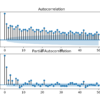# Search results for "regression"## A Gentle Introduction to Linear Regression With Maximum Likelihood Estimation

Linear regression is a classical model for predicting a numerical quantity. The parameters of a linear regression model can be estimated using a least squares procedure or by a maximum likelihood estimation procedure. Maximum likelihood estimation is a probabilistic framework for automatically finding the probability distribution and parameters that best describe the observed data. Supervised […]## Autoregression Forecast Model for Household Electricity Consumption

Given the rise of smart electricity meters and the wide adoption of electricity generation technology like solar panels, there is a wealth of electricity usage data available. This data represents a multivariate time series of power-related variables that in turn could be used to model and even forecast future electricity consumption. Autocorrelation models are very […]

## How can I change a neural network from “regression” to “classification”?

A How can I change a neural network from “regression” to “classification”? A neural network designed for a regression problem can easily be changed to classification. It requires two changes to the code: A change to the output layer. A change to the loss function. A neural network designed for regression will likely have an […]

## What is the difference between “classification” and “regression”?

A What is the difference between “classification” and “regression”? Classification involves assigning an observation a label. Some examples include: Assigning an email a label of “spam” or “not spam”. Assigning a credit card transaction a label of “fraud” or “ok”. Assigning a medical record a label of “healthy” or “sick”. Regression involves predicting a numerical […]

## How do I calculate accuracy for regression?

A How do I calculate accuracy for regression? You can’t. Accuracy is a measure for classification. You calculate the error for regression. Learn more here: Difference Between Classification and Regression in Machine Learning Permalink## How to Solve Linear Regression Using Linear Algebra

Linear regression is a method for modeling the relationship between one or more independent variables and a dependent variable. It is a staple of statistics and is often considered a good introductory machine learning method. It is also a method that can be reformulated using matrix notation and solved using matrix operations. In this tutorial, […]## Difference Between Classification and Regression in Machine Learning

There is an important difference between classification and regression problems. Fundamentally, classification is about predicting a label and regression is about predicting a quantity. I often see questions such as: How do I calculate accuracy for my regression problem? Questions like this are a symptom of not truly understanding the difference between classification and regression […]## Autoregression Models for Time Series Forecasting With Python

Autoregression is a time series model that uses observations from previous time steps as input to a regression equation to predict the value at the next time step. It is a very simple idea that can result in accurate forecasts on a range of time series problems. In this tutorial, you will discover how to […]## How To Implement Logistic Regression From Scratch in Python

Logistic regression is the go-to linear classification algorithm for two-class problems. It is easy to implement, easy to understand and gets great results on a wide variety of problems, even when the expectations the method has of your data are violated. In this tutorial, you will discover how to implement logistic regression with stochastic gradient […]## How to Implement Linear Regression From Scratch in Python

The core of many machine learning algorithms is optimization. Optimization algorithms are used by machine learning algorithms to find a good set of model parameters given a training dataset. The most common optimization algorithm used in machine learning is stochastic gradient descent. In this tutorial, you will discover how to implement stochastic gradient descent to […]